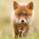10910 vues
Update to the zigzag , now it ignores the second top/bottom untill it finds a inversed top/bottom. TBTBTBTBTBTBTB instead of TBBTTBTBBTBTTB.

Updated to V8, link bellow in the related ideas.
```study(title="[RS]Fractals V5", overlay=true)
//  ||---   V1: Added filtering option
//  ||---   V4: diferentiated Bill Williams fractals and regular fractals as a choice
//  ||---   V5: modified zigzag
//  ||---   Fractal Recognition:
filterBW = input(false, title="filter Bill Williams Fractals:")
filterFractals = input(false, title="Filter fractals using extreme method:")
length = input(24, title="Extreme Window:")

regulartopfractal = high < high and high < high and high > high and high > high
regularbotfractal = low > low and low > low and low < low and low < low

billwtopfractal = filterBW ? false : (high < high and high < high and high > high and high > high ? true : false)
billwbotfractal = filterBW ? false : (low > low and low > low and low < low and low < low ? true : false)

ftop = filterBW ? regulartopfractal : regulartopfractal or billwtopfractal
fbot = filterBW ? regularbotfractal : regularbotfractal or billwbotfractal

topf = ftop ? high >= highest(high, length) ? true : false : false
botf = fbot ? low <= lowest(low, length) ? true : false : false

filteredtopf = filterFractals ? topf : ftop
filteredbotf = filterFractals ? botf : fbot
//  ||------------------------------------------------------------------------------------------------------

plotshape(filteredtopf, style=shape.triangledown, location=location.abovebar, color=red, text="•", offset=-2)
plotshape(filteredbotf, style=shape.triangleup, location=location.belowbar, color=lime, text="•", offset=-2)
//  ||---   V1 : Added Swing High/Low Option
ShowSwingsHL = input(true)
highswings = filteredtopf == false ? na : valuewhen(filteredtopf == true, high, 2) < valuewhen(filteredtopf == true, high, 1) and valuewhen(filteredtopf == true, high, 1) > valuewhen(filteredtopf == true, high, 0)
lowswings = filteredbotf == false ? na : valuewhen(filteredbotf == true, low, 2) > valuewhen(filteredbotf == true, low, 1) and valuewhen(filteredbotf == true, low, 1) < valuewhen(filteredbotf == true, low, 0)
//---------------------------------------------------------------------------------------------------------
//  ||---   Offset calculation:
//  ||--- unable to use, plots cant use series for offset value...
//hsoffset = n-valuewhen(ftop == true, n, 1)
//lsoffset = n-valuewhen(fbot == true, n, 1)
//---------------------------------------------------------------------------------------------------------
plotshape(ShowSwingsHL ? highswings : na, style=shape.triangledown, location=location.abovebar, color=maroon, text="H", offset=-2)
plotshape(ShowSwingsHL ? lowswings : na, style=shape.triangleup, location=location.belowbar, color=green, text="L", offset=-2)
//  ||---   V2 : Plot Lines based on the fractals.
showchannel = input(true)
plot(showchannel ? (filteredtopf ? high : na) : na, color=black, offset=-2)
plot(showchannel ? (filteredbotf ? low : na) : na, color=black, offset=-2)
//---------------------------------------------------------------------------------------------------------
//  ||---   HLswings channel: unable to offset values
//plot(showchannel ? (highswings ? high : na) : na, color=black, offset=-2)
//plot(showchannel ? (lowswings ? low : na) : na, color=black, offset=-2)
//----------------------------------------------------------------------------------------------------------
//  ||---   ZigZag:
showZigZag = input(true)

istop = filteredtopf ? true : false
isbot = filteredbotf ? true : false

zigzag = not showZigZag ? na : (
istop and topcount > botcount ? high :
isbot and topcount < botcount ? low :
na )

//zigzag = not showZigZag ? na : ( filteredtopf == true ? high : filteredbotf == true ? low : na )
plot(zigzag, color=black, offset=-2)

```

## Commentairesjacxia81
@jacxia81, its possible only as a numeric variable plot (it shows after the indicators name on the top left of the chart), or another indicator just for that purpose
Répondre